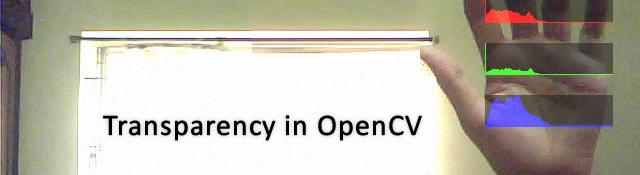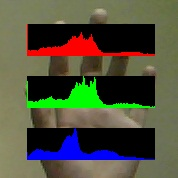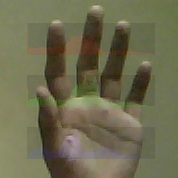## Transparent image overlays in OpenCV

Time for some fun! Today we'll be creating an interesting program today. I'll be referring to a few old posts I've done. The the final result will be you'll see histograms of R, G and B on top of a live video feed. And they will be semi  transparent. Okay, so lets get started with this!

## Prerequisites

For now, I'll assume you've read these posts:

## The main function

Lets get to the code now. Create a new project. First, add the standard OpenCV headers and the videoInput headers:

```#include <cv.h>
#include <highgui.h>
#include "videoInput.h"
```

Then, the main function:

```void main()
{
```

I'll use the videoInput method. It works on my computer. The functions of the internal libraries of OpenCV don't work with my webcam for some reason (know why? let me know please).

```    videoInput VI;
int numDevices = VI.listDevices();

int device1= 0;

// Setup the capture
VI.setupDevice(device1);
int width = VI.getWidth(device1);
int height = VI.getHeight(device1);
```

Then, we create an image that will hold the webcam's live feed:

```    IplImage* img= cvCreateImage(cvSize(width, height), 8, 3);
unsigned char* yourBuffer = new unsigned char[VI.getSize(device1)];
```

img will hold the image in OpenCV's format. yourBuffer holds the image in videoInput format (raw bytes). We'll be creating live histograms for the image, so we initialize a histogram structure.

```    // Variables to be used for the histgoram
int numBins = 256;
float range[] = {0, 255};

float *ranges[] = { range };

// Initialize a histogram
CvHistogram *hist = cvCreateHist(1, &numBins, CV_HIST_ARRAY, ranges, 1);
cvClearHist(hist);
```

We're creating a histogram with 256 bins, it is uniform and is one dimensional.

Next, we create a handy black image. You'll see why this is needed in a minute:

```    IplImage* imgBlack = cvCreateImage(cvSize(128, 32), 8, 1);
cvZero(imgBlack);
```

Then we create some temporary images we'll be needing:

```    IplImage* imgRed = cvCreateImage(cvGetSize(img), 8, 1);
IplImage* imgGreen = cvCreateImage(cvGetSize(img), 8, 1);

IplImage* imgBlue = cvCreateImage(cvGetSize(img), 8, 1);

IplImage* imgRedHist = cvCreateImage(cvSize(128, 32), 8, 3);
IplImage* imgGreenHist = cvCreateImage(cvSize(128, 32), 8, 3);
IplImage* imgBlueHist = cvCreateImage(cvSize(128, 32), 8, 3);
```

The first three images will hold the red, green and blue of the captured frame. The next three images will hold the histograms.

Now we get into the loop. This loop constantly grabs new frames from your camera:

```    while(1)
{
VI.getPixels(device1, yourBuffer, false, false);
img->imageData = (char*)yourBuffer;
cvConvertImage(img, img, CV_CVTIMG_FLIP);
```

The first line actually grabs the raw bytes. The second line associates these bytes with the OpenCV structure. Finally, we flip the image (because the image captured is upside down).

We split the image into constituent channels next:

```        cvSplit(img, imgBlue, imgGreen, imgRed, NULL);
```

Then, as in the Drawing Histograms in OpenCV post, we draw histograms for each channel:

```        cvCalcHist(&imgRed, hist, 0, 0);              // Calculate the histogram for red
IplImage* imgHistRedOnly = DrawHistogram(hist, 0.5, 0.5);    // Draw it

cvClearHist(hist);                            // Clear the histgoram

cvCalcHist(&imgGreen, hist, 0, 0);            // Reuse it to calculate histogram for green
IplImage* imgHistGreenOnly = DrawHistogram(hist, 0.5, 0.5);

cvClearHist(hist);

cvCalcHist(&imgBlue, hist, 0, 0);            // And again for blue
IplImage* imgHistBlueOnly = DrawHistogram(hist, 0.5, 0.5);
cvClearHist(hist);
```

Next, we zero out the previous frame's histogram:

```        cvZero(imgRedHist);
cvZero(imgGreenHist);
cvZero(imgBlueHist);
```

Then, we merge the current histogram image into a 3 channel image. The DrawHistogram returns a single channel image. But because we want to overlay it onto a 3 channel image, we need to convert it into a 3 channel image:

```        cvMerge(imgHistBlueOnly, imgBlack, imgBlack, NULL, imgBlueHist);
cvMerge(imgBlack, imgHistGreenOnly, imgBlack, NULL, imgGreenHist);
cvMerge(imgBlack, imgBlack, imgHistRedOnly, NULL, imgRedHist);
```

This is where the imgBlack images are useful. We use the histogram image (the one we got from DrawHistogram), put it into the appropriate channel and set the other channels to zero.

Finally, we overlay the images with transparency:

```        OverlayImage(img, imgRedHist, cvPoint(485, 24), cvScalar(0.5,0.5,0.5,0.5), cvScalar(0.5,0.5,0.5,0.5));
OverlayImage(img, imgGreenHist, cvPoint(485, 76), cvScalar(0.5,0.5,0.5,0.5), cvScalar(0.5,0.5,0.5,0.5));
OverlayImage(img, imgBlueHist, cvPoint(485, 128), cvScalar(0.5,0.5,0.5,0.5), cvScalar(0.5,0.5,0.5,0.5));
```

We'll get to this function in sometime. Those super long parameters actually mean something. And you'll know what they mean when we get to it.

And finally, we display the image:

```        cvShowImage("Overlay", img);
```

Then, we wait for the user to press escape. If he does, we quit. Otherwise, the loop continues. Thus the main function ends.

```        if(cvWaitKey(15)==27) break;
}
}
```

## Drawing histograms

Here's the function to draw histograms. Just in case:

```// A function to draw the histogram
IplImage* DrawHistogram(CvHistogram *hist, float scaleX=1, float scaleY=1)
{
// Find the maximum value of the histogram to scale
// other values accordingly
float histMax = 0;

cvGetMinMaxHistValue(hist, 0, &histMax, 0, 0);

// Create a new blank image based on scaleX and scaleY
IplImage* imgHist = cvCreateImage(cvSize(256*scaleX, 64*scaleY), 8 ,1);

cvZero(imgHist);

// Go through each bin
for(int i=0;i<255;i++)
{
float histValue = cvQueryHistValue_1D(hist, i);        // Get value for the current bin...

float nextValue = cvQueryHistValue_1D(hist, i+1);    // ... and the next bin

// Calculate the four points of the polygon that these two
// bins enclose
CvPoint pt1 = cvPoint(i*scaleX, 64*scaleY);
CvPoint pt2 = cvPoint(i*scaleX+scaleX, 64*scaleY);
CvPoint pt3 = cvPoint(i*scaleX+scaleX, (64-nextValue*64/histMax)*scaleY);

CvPoint pt4 = cvPoint(i*scaleX, (64-histValue*64/histMax)*scaleY);

// A close convex polygon
int numPts = 5;

CvPoint pts[] = {pt1, pt2, pt3, pt4, pt1};

// Draw it to the image
cvFillConvexPoly(imgHist, pts, numPts, cvScalar(255));

}

// Return it... make sure you delete it once you're done!
return imgHist;
}
```

I've gone through this function in detail in the Drawing Histograms in OpenCV post.

## Creating Overlays

Now we get to the OverlayImage function. Start by creating the first line:

```void OverlayImage(IplImage* src, IplImage* overlay, CvPoint location, CvScalar S, CvScalar D)

{
```

This function takes the source image (src) and puts the image overlay on top of it. location is the position where the image must be put. S and D are blending coefficients.

When overlaying, you're obviously replacing pixel values by other values. These values are computed by multiplying S and the RGB values at the source and adding to D*RGB Values in the overlay image.

To do this, we must iterate over the entire image to be overlaid:

```    for(int x=0;xwidth;x++)
{
if(x+location.x>=src->width) continue;
for(int y=0;yheight;y++)
{
if(y+location.y>=src->height) continue;
```

The if statements keep the loops from going beyond the source image. Then, we get the pixel value at (x,y) in both src _and _overlay.

```            CvScalar source = cvGet2D(src, y+location.y, x+location.x);
CvScalar over = cvGet2D(overlay, y, x);
```

And we calculate the new "merged" pixel value:

```            CvScalar merged;
for(int i=0;i<4;i++)
merged.val[i] = (S.val[i]*source.val[i]+D.val[i]*over.val[i]);
```

We set this as the next pixel value and that ends the function!

```            cvSet2D(src, y+location.y, x+location.x, merged);
}
}
}
```

Because src is a pointer, any changes made in the image will automatically be reflected in the main function. So we don't need to return anything.

## Done!

After linking the appropriate library files (Project Properties -> Configuration Properties -> Linker -> Input) for OpenCV and videoInput, the program should compile. On running you'll see live video and a histogram on the right.

Note: I assume that your webcam/camera will work at 640x480. If it doesn't make sure you change the location parameter (I use cvPoint(485, 20), etc in this post).

## Play around with S and D

Different sets of S and D produce different results. Here are a few that results that I got:Standard 50% transparency; S = (0.5, 0.5, 0.5, 0.5) D = (0.5, 0.5, 0.5, 0.5)Black turns see through; S = (1,1,1,1) D = (1,1,1,1)No transparency. Simple copy paste; S = (0,0,0,0) D = (1,1,1,1)10% transparent; S = (0.1, 0.1, 0.1, 0.1) D = (0.9, 0.9, 0.9, 0.9)90% transparent; S = (0.9, 0.9, 0.9, 0.9) D = (0.1, 0.1, 0.1, 0.1)

Do you see a pattern? If you want x% transparency, you set S to x and D to 1-x. And try negative values too ;) Did you get some interesting results?

#### Related posts#### 7 unique neural network architectures#### Connected Component Labelling

Utkarsh Sinha created AI Shack in 2010 and has since been working on computer vision and related fields. He is currently at Microsoft working on computer vision.